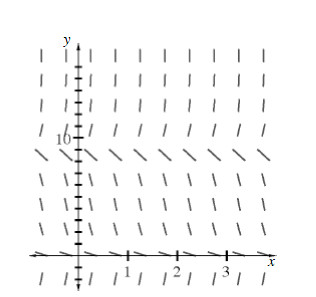### Home > CALC > Chapter 10 > Lesson 10.1.8 > Problem10-83

10-83.

Examine the slope field shown below. Imagine drawing some solutions, beginning with a variety of $y$-intercepts above the $x$-axis. What will be $\lim\limits_ { x \rightarrow \infty } y$  for each of the solutions?Where are the possible horizontal asymptotes for the solution curve?